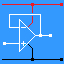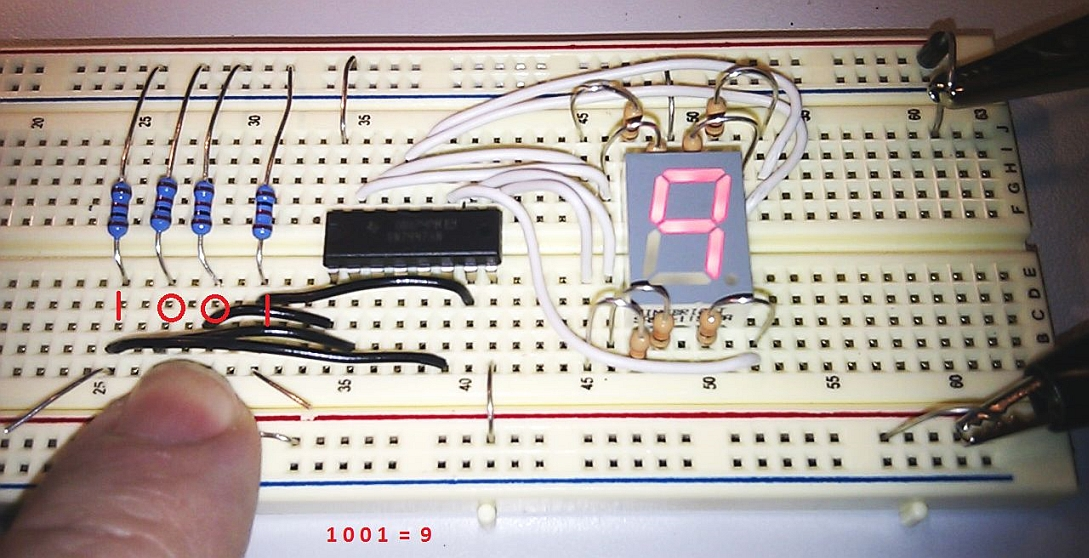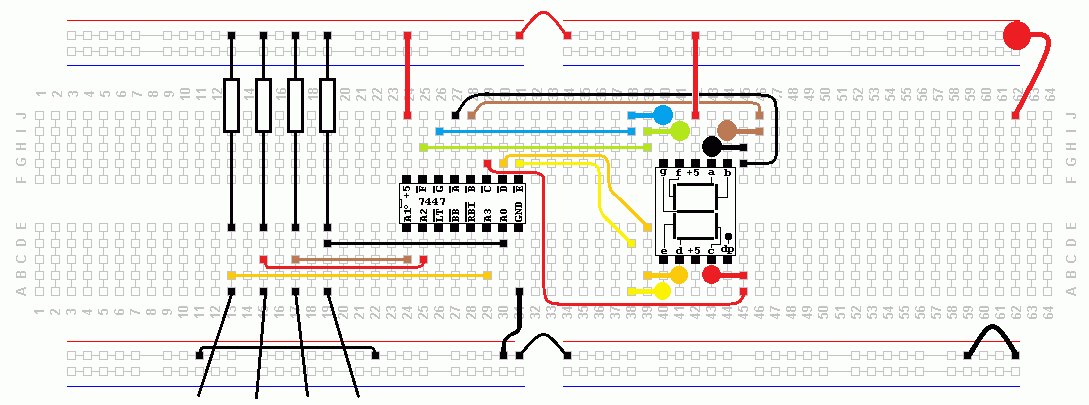RANDOM PAGE

SITE SEARCH

LOG
IN

HELP

# Binary Coded Decimal

This is the AQA version closing after June 2019. Visit the the version for Eduqas instead.

a

## The BCD Number System

This is used in digital clocks, calculators, cash registers and any device that only ever displays digits between 0 and 9.

A four bit binary number is used to represent each decimal digit.

A number like 32 is represented as two four-bit digits - The three is converted into 0011 and the two is converted into 0010.

In pure binary 32 would be represented as 100000.

The table below shows the bit patterns for each digit between 0 and 9.

 Decimal Binary 0 0000 1 0001 2 0010 3 0011 4 0100 5 0101 6 0110 7 0111 8 1000 9 1001

Here is a circuit that converts a BCD digit into seven signals that light the LEDs on a seven segment display. Here the binary input is 0011 (3 in decimal) and suitable segments are lit on the display. The lamp test LT pin lights all seven segments and the decimal point for test purposes. RBI and BI/BRO are used to blank unwanted zeros on multi digit displays so instead of seeing 000123 you just see 123. Google for the data sheet for full details.• The circuit below runs on 5 Volts.
• The seven LED current limiting resistors are 330 Ω
• The four pull-up resistors are 1 KΩ• The finger in the image is pressing two wires, connecting them to zero.
• The other two wires are at logic level ONE because of the pull-up resistors.
• 1 0 0 1 = 9• There are four improvised switches bottom left.
• There is a polarity protection diode top-right.
• It is a good idea to colour code the wiring.
• Very few wires cross over each other. This makes fault finding easier.
b

## Geek BCD Clock

It's possible to build a clock that outputs the time in Binary Coded Decimal. It uses a row of LEDs to show the hours (red) and the minutes (green).1. Work out what the time is ...
2. Why are the LEDs grouped in 2, 4, 3, 4?

reviseOmatic V3     Contacts, ©, Cookies, Data Protection and Disclaimers Hosted at linode.com, London